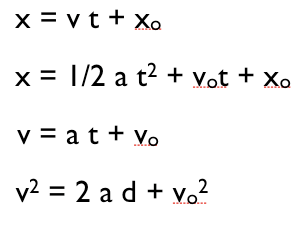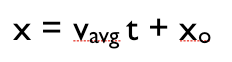## Tuesday, April 12, 2011

### Kinematics: Is Average Velocity Queen?

In college physics, students often learn a slew of kinematic equations:I'm a big fan of only teaching this one:There are four reasons I like just using this, both personally and for teaching. It's all-purpose; conundrum-focused; tool-and-representation-infused; and intuition-refining.

First: The equation works all the time. There are no caveats to remember. As I've gotten older, this is the first of my two "go-to" ideas in kinematics. I will say that I almost never use the 2nd or 4th equation from above to solve any real or textbook problem.

Second: You get to engage students with the conundrums of figuring out what the average velocity is for a variety of situations: unchanging velocity, steadily changing velocity, piece-wise changing, non-linear changing, etc.

Third: You can explore various techniques like weighted averages or riemann-sums using data from tables, graphs, and other representations. It naturally builds in ideas of approximation.

Fourth: With these tools, you get to explore the boundaries of intuition (e.g., looking at this graph, what would you guess the average velocity to be? Or the famous example of determining the average speed when traveling 45 mph one way and 60 mph the other way on a round trip)

This is me guessing what the average velocity should be for a few situations:At the end of the day, for instruction, you are likely to still have to fold in ideas about instantaneous velocity, changing velocity, and acceleration. It's something I want to talk about, but I'm going to leave it for another post.

1.My colleague calls those equations "The Big Four" and I find my advanced students referring to them all the time if they have him for intro. I get pretty frustrated that they don't remember the assumptions needed for them to be true, especially the second one (constant acc). But I have to say that the 4th one is my biggest pet peeve. I can't believe that texts introduce it before introducing energy. It's so easy once you've learned about KE and gravitational PE, and also you can see it's limitations.

I like how you describe the queen but I have to say my initial visceral reaction was "but what about the physics behind why the velocity changes?" I guess you need the king ;)

2.Yeah, I know a lot of people who are unhappy with the 4th one because it's really just energy conservation. But I don't like the 2nd one either. I'm OK thinking about it in terms of taylor expansions, where the first term is the correction term (i.e., how far off would your answer be if you ignored acceleration?) If 1/2at^2 is really small over some time interval, then by all means use the constant velocity equation; if it's not small, then figure out what the average velocity was for that time interval.

3.Brian,

This is great. I don't like the big four either. But we draw velocity-time graphs for solving most kinematic problems. A v-t graph has everything you need: velocities (obviously), displacement (area) and acceleration (slope). Once in a while there is a tricky problem where we'll have to write 2 equations with 2 unknowns (area eq. and slope eq.) to find what we need. While my students emerge with an awesome understanding of kinematic graphs, I often wonder if doing it this way is still another exercise in algorithms rather than understanding. What do you think?

Your use of the average velocity method seems to stress understanding, but, again, does it ever journey into equation abuse?

4.My students seem to like the comfort of the "accounting" system of 5 variables and 5 equations. Each equation can be viewed through the lens of multiple concepts, but they can all be derived algebraicaly/geometrically from that average velocity formula:

5.Frank, I think there is no fully escaping the algorithm trap. With that said, that's why I want to spend a fair amount of time on developing this one idea--average velocity. For that idea, everything else is about finding a smart algorithm to help you figure out what that average velocity is. I want to let the algorithms serve the concept, not the other way around, whereby most students see the algorithm as defining the concept.

Ed, I hear what you're saying, but I don't really want to use the equation to derive other equations, at least not for students. I did that for years. It was a nice exercise for me, but it's not been helpful for students, at least in my experience. I find the only people who appreciate those things are people who already understand what you are doing and why.

6.Thanks, Brian. So it sounds like my graphical approach is OK then, b/c students are reasoning through the graph and finding an algorithm that fits the graph concept, right?

7.Frank, I think graphical approaches are great! What I hear from you is your possible concern that you are just replacing a "plug-n-chug" algorithm with a "do-this-with-graphs" algorithm. Is that right? While that's possible, I think it's unlikely knowing everything else about what you are doing in your classroom.

For one, I think the use of multiple representations–as you obviously you do in your classroom–are a good antidote. Two, I think it's important to engage students in problems that continue to press the boundaries of what they know and don't know about the concepts. Algorithms can't do all the work in novel problems, when a sense of concept is required.

8.When analyzing the displacement of an object undergoing acceleration, I have called the 'average velocity' the 'velocity that an object should have if it were to accomplish the same displacement at a constant velocity'.

9.Sam, in that sense, I like to call it the "equivalent" velocity... rather than "average".

10.Ooooh. The "equivalent constant velocity." I REALLY like that phrase!

I've used the "ghost racer" analogy for average velocity. Like if you're playing Mario Cart, how fast a ghost racer traveling at constant speed would have to be to tie you at the finish line.

The big trap with "average velocity" with my students is AVERAGE. Immediately the want to start adding up velocities and dividing. Or they are stumped when I give them just displacement and time with nothing to "average."

So I really like "equivalent." Does anyone have a problem with unconventional terminology in a class for non-physics majors? (I personally don't.)

11.The ghost racer is nice. All and all, the concept is a bit odd it because involves a hypothetical, "the constant velocity one would need to have if..."

And, yeah, I don't have a problem with changing terminology, e.g. interaction energy over potential energy.

12.Brian,
When would you use 'average' velocity instead of 'equivalent'?

13.This is a good post. This post gives truly quality information. I’m definitely going to look into it. Really very useful tips are provided here. Thank you so much. Keep up the good works. sales velocity formula